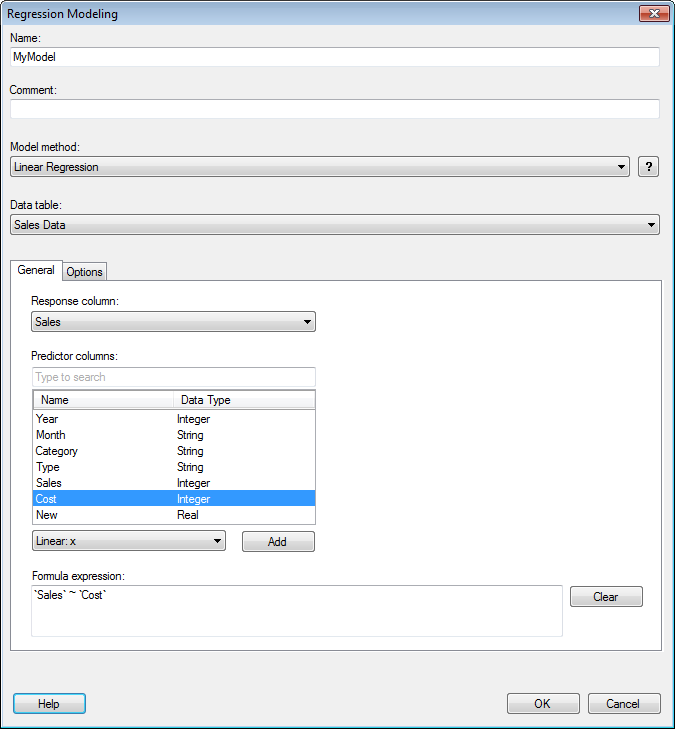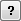﻿ Details on Regression Modeling – General

# Details on Regression Modeling – General

This tool allows you to create regression models using the TIBCO Enterprise Runtime for R engine, without the need of writing any scripts yourself. A model page will be created (see The Model Page) and the model will be added to the Analytic Models panel.

• To reach the Regression Modeling dialog:

2. Select Regression Modeling....Option Description Name The name of the model as you want it to be referenced in the Analytic Models panel. Comment A field for optional comments on the model. It is recommended that the comment field describes what type of data you should use this model for. Model method Specifies the prediction model method. Choose from Linear Regression and Regression Tree.Displays more information about the currently selected model method. Data table Specifies the data table on which the model will be calculated. Response column Specifies what you are trying to predict. The selected response column is automatically added to the Formula expression field. Numeric columns in the selected data table are available for selection. However, note that currency columns are converted to real, which can result in a lack of precision in the modeling. Predictor columns Lists all columns in the selected data table that can be used as predictor columns. Note that currency columns are converted to real, which can result in a lack of precision in the modeling. Select all variables that you think might affect the values you are trying to predict (the selected response column) and click Add after specifying a suitable transformation. [Drop-down list] Select a transformation from this list to apply it to the selected predictor columns before clicking Add and sending the predictor columns to the Formula expression field. The  selected method should reflect the expected relationship between the response column and the selected predictor column. For example, if the response column is expected to linearly depend on another column, then this column should be added as a predictor column using the Linear: x method. If the relationship between the two columns instead is logarithmic, then you might add the predictor column using the Log: log(x) method. Add Adds the selected predictor columns to the Formula expression field using the transformation selected in the drop-down list. Formula expression Displays the relationship set up between the response column and the selected predictor columns. You can also edit the formula expression field manually if you want to create more advanced expressions. Clear Clears the Formula expression field.

Details on Regression Modeling – Options